# Generalizations of Caristi Kirk's Theorem on Partial Metric Spaces

## Abstract

In this article, lower semi-continuous maps are used to generalize Cristi-Kirk's fixed point theorem on partial metric spaces. First, we prove such a type of fixed point theorem in compact partial metric spaces, and then generalize to complete partial metric spaces. Some more general results are also obtained in partial metric spaces.

2000 Mathematics Subject Classification 47H10,54H25

## 1. Introduction and preliminaries

In 1992, Matthews [1, 2] introduced the notion of a partial metric space which is a generalization of usual metric spaces in which d(x, x) are no longer necessarily zero. After this remarkable contribution, many authors focused on partial metric spaces and its topological properties (see, e.g. -)

Let X be a nonempty set. The mapping p : X × X → [0, ∞) is said to be a partial metric on X if for any x, y, z X the following conditions hold true:

(PM1) p(x, y) = p(y, x) (symmetry)

(PM2) If p(x, x) = p(x, y) = p(y, y) then x = y (equality)

(PM3) p(x, x) ≤ p(x, y) (small self-distances)

(PM4) p(x, z) + p(y, y) ≤ p(x, y) + p(y, z) (triangularity)

for all x, y, z X. The pair (X, p) is then called a partial metric space(see, e.g. [1, 2]). We use the abbreviation PMS for the partial metric space (X, p).

Notice that for a partial metric p on X, the function d p : X × X → [0, ∞) given by(1.1)

is a (usual) metric on X. Observe that each partial metric p on X generates a T0 topology τp on X with a base of the family of open p-balls {B p (x, ε): x X, ε > 0}, where B p (x, ε) = {y X : p(x, y) < p(x, x) + ε} for all x X and ε > 0. Similarly, closed p-ball is defined as B p [x, ε] = {y X : p(x, y) ≤ p(x, x) + ε}

Definition 1. (see, e.g. [1, 2, 6])

(i) A sequence {x n } in a PMS (X, p) converges to x X if and only if p(x, x) = limn→∞p(x, x n ),

(ii) a sequence {x n } in a PMS (X, p) is called Cauchy if and only if limn,m→∞p(x n , x m ) exists (and finite),

(iii) A PMS (X, p) is said to be complete if every Cauchy sequence {x n } in X converges, with respect to τ p , to a point x X such that p(x, x) = limn,m→∞p(x n , x m ).

(iv) A mapping f : XX is said to be continuous at x0 X, if for every ε > 0, there exists δ > 0 such that f(B(x0, δ)) B(f(x0), ε).

Lemma 2. (see, e.g. [1, 2, 6])

(A) A sequence {x n } is Cauchy in a PMS (X, p) if and only if {x n } is Cauchy in a metric space (X, d p ),

(B) A PMS (X, p) is complete if and only if a metric space (X, d p ) is complete. Moreover,(1.2)

## 2. Main Results

Let (X, p) be a PMS, c X and φ : C+ a function on C. Then, the function φ is called a lower semi-continuous (l.s.c) on C whenever(2.1)

Also, let T : XX be an arbitrary self-mapping on X such that(2.2)

where T is called a Caristi map on (X, p).

The following lemma will be used in the proof of the main theorem.

Lemma 3. (see, e.g. [8, 7]) Let (X, p) be a complete PMS. Then

1. (A)

If p(x, y) = 0 then x = y,

2. (B)

If xy, then p(x, y) > 0.

Proof. Proof of (A). Let p(x, y) = 0. By (PM3), we have p(x, x) ≤ p(x, y) = 0 and p(y, y) ≤ p(x, y) = 0. Thus, we haveHence, by (PM2), we have x = y.

Proof of (B). Suppose xy. By definition p(x, y) ≥ 0 for all x, y X. Assume p(x, y) = 0. By part (A), x = y which is a contradiction. Hence, p(x, y) > 0 whenever xy.

Lemma 4. (see, e.g. [8, 7]) Assume x n z as n → ∞ in a PMS (X, p) such that p(z, z) = 0. Then, limn→∞p(x n , y) = p(z, y) for every y X.

Proof. First, note that lim n→∞p(x n , z) = p(z, z) = 0. By the triangle inequality, we haveandHence,Letting n → ∞ we conclude our claim. □

The following theorem is an extension of the result of Caristi (; Theorem 2.1)

Theorem 5. Let (X, p) be a complete PMS, φ : X+a lower semi-continuous (l. s.c) function on X. Then, each self-mapping T : XX satisfying (2.2) has a fixed point in X.

Proof. For each x X, define(2.3)

Since x S(x), then S(x) ≠ . From (2.3), we have 0 ≤ α (x) ≤ φ(x).

Take x X. We construct a sequence {x n } in the following way:(2.4)

Thus, one can easily observe that(2.5)

Note that (2.5) implies that {φ(x n )} is a decreasing sequence of real numbers, and it is bounded by zero. Therefore, the sequence {φ(x n )} is convergent to some positive real number, say L. Thus, regarding (2.5), we have(2.6)

From (2.5) and (2.6), for each k , there exists N k such that(2.7)

Regarding the monotonicity of {φ(x n )}, for mnN k , we have(2.8)

Thus, we obtain(2.9)

On the other hand, taking (2.5) into account, together with the triangle inequality, we observe that(2.10)

Analogously,(2.11)

By induction, we obtain that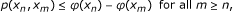(2.12)

and taking (2.9) into account, (2.12) turns into(2.13)

Since the sequence {φ(x n )} is convergent which implies that the right-hand side of (2.13) tends to zero. By definition,(2.14)

Since p(x n , x m ) tends to zero as n, m → ∞, then (2.14) yields that {x n } is Cauchy in (X, d p ). Since (X, p) is complete, by Lemma 2, (X, d p ) is complete, and thus the sequence {x n } is convergent in X, say z X. Again by Lemma 2,(2.15)

Since limn,m→∞p(x n , x m ) = 0, then by (2.15), we have p(z, z) = 0.

Because φ is l.s.c together with (2.13)(2.16)

and thusBy definition, z S(x n ) for all n and thus α(x n ) ≤ φ(z). Taking (2.6) into account, we obtain Lφ (z). Moreover, by l.s.c of φ and (2.6), we have φ (z) limn→∞φ (x n ) = L. Hence, φ (z) = L.

Since z S(x n ) for each n and (2.2), then Tz S(z) and by triangle inequality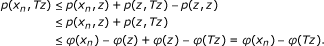is obtained. Hence, Tz S(x n ) for all n which yields that α(x n ) ≤ φ(Tz) for all n .

From (2.6), the inequality φ(Tz) ≥ L is obtained. By φ (Tz) ≤ φ (z), observed by (2.2), and by the observation φ (z) = L, we achieve as follows: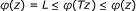Hence, φ(Tz) = φ (z). Finally, by (2.2), we have p(Tz, z) = 0. Regarding Lemma 3, Tz = z.

The following theorem is a generalization of the result in 

Theorem 6. Let φ : X+be a l.s.c function on a complete PMS. If φ is bounded below, then there exits z X such thatProof. It is enough to show that the point z, obtained in the Theorem 5, satisfies the statement of the theorem. Following the same notation in the proof of Theorem 5, it is needed to show that x S(z) for xz. Assume the contrary, that is, for some wz, we have w S(z). Then, 0 < p(z, w) ≤ φ(z) - φ (w) implies φ (w) < φ (z) = L. By triangular inequality,which implies that w S(x n ) and thus α(x n ) ≤ φ(w) for all n . Taking the limit when n tends to infinity, one can easily obtain Lφ (w), which is in contradiction with φ (w) < φ (z) = L. Thus, for any x X, xz implies x S(z) that is,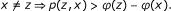Theorem 7. Let X and Y be complete partial metric spaces and T : XX an self-mapping. Assume that R : XY is a closed mapping, φ : X+is a l.c.s, and a constant k > 0 such that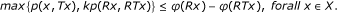(2.17)

Then, T has a fixed point.

Proof. For each x X, we define(2.18)

For x X set x1 : = x and construct a sequesnce x1, x2, x3, ..., x n , ... as in the proof of Theorem 5:

xn+1 S(x n ) such thefor each n .

As in Theorem 5, one can easily get that {x n } is convergent to z X. Analogously, {Rx n } is Cauchy sequence in Y and convergent to some t. Since R is closed mapping, Rz = t. Then, as in the proof of Theorem 5, we haveAs in the proof of Theorem 6, we get that xz implies x S(z). From (2.17), Tz S(z), we have Tz = z.

Define p x : XR+ such that p x (y) = p(x, y).

Theorem 8. Let (X, p) be a complete PMS. Assume for each x X, the function p x defined above is continuous on X, and is a family of mappings f : XX. If there exists a l.s.c function φ : X+such that(2.19)

then, for each x X, there is a common fixed point z of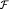such thatProof. Let S(x): = {y X : p(x, y) ≤ φ(x) - φ (y)} and α(x): = inf{φ (y): y S(x)} for all x X. Note that x S(x), and so S(x) ≠ as well as 0 ≤ α (x) ≤ φ(x).

For x X, set x1 := x and construct a sequence x1, x2, x3, ..., x n , ... as in the proof of Theorem 5: xn+1 S(x n ) such that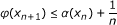for each n . Thus, one can observe that for each n,

(i) p(x n , xn+1) ≤ φ(x n ) - φ(xn+1).

(ii).

Similar to the proof of Theorem 5, (ii) implies that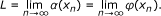(2.20)

Also, using the same method as in the proof of Theorem 5, it can be shown that {x n } is a Cauchy sequence and converges to some z X and φ(z) = L.

We shall show that f(z) = z for all. Assume on the contrary that there issuch that f(z) ≠ z. Replace x = z in (2.19); then we get φ(f(z)) < φ (z) = L:

Thus, by definition of L, there is n such that φ (f(z)) < α(x n ). Since z S(x n ), we havewhich implies that f(z) S(x n ). Hence, α(x n ) ≤ φ(f(z)) which is in a contradiction with φ (f(z)) < α(x n ). Thus, f(z) = z for all.

Since z S(x n ), we have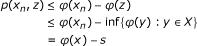is obtained. □

The following theorem is a generalization of (; Theorem 2.2).

Theorem 9. Let A be a set, (X, p) as in Theorem 8, g : AX a surjective mapping and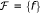a family of arbitrary mappings f : AX. If there exists a l.c.s: function φ : X → [0, ∞) such that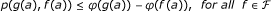(2.21)

and each a A, then g andhave a common coincidence point, that is, for some b A; g(b) = f(b) for all.

Proof. Let x be arbitrary and z X as in Theorem 8. Since g is surjective, for each x X there is some a = a(x) such that g(a) = x. Letbe a fixed mapping. Define by f a mapping h = h(f) of X into itself such that h(x) = f(a), where a = a(x), that is, g(a) = x. Letbe a family of all mappings h = h(f). Then, (2.21) yields that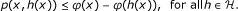Thus, by Theorem 8, z = h(z) for all. Hence g(b) = f(b) for all, where b = b(z) is such that g(b) = z.

Example 10. Let X = +and p(x, y) = max{x, y}; then (X, p) is a PMS (see, e.g. .) Suppose T : XX such that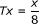for all x X and ϕ(t): [0, ∞) → [0, ∞) such that ϕ (t) = 2t. Then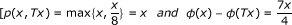Thus, it satisfies all conditions of Theorem 5. it guarantees that T has a fixed point; indeed x = 0 is the required point.

## References

1. 1.

Matthews SG: Partial metric topology. Research Report 212. Department of Computer Science, University of Warwick 1992.

2. 2.

Matthews SG: Partial metric topology. General Topology and its Applications. Proceedings of the 8th Summer Conference, Queen's College (1992). Ann NY Acad Sci 1994, 728: 183–197.

3. 3.

Oltra S, Valero O: Banach's fixed point theorem for partial metric spaces. Rendiconti dell'Istituto di Matematica dell'Universit di Trieste 2004,36(1–2):17–26.

4. 4.

Valero O: On Banach fixed point theorems for partial metric spaces. Appl Gen Topol 2005, 62: 229–240.

5. 5.

Altun I, Sola F, Simsek H: Generalized contractions on partial metric spaces. Topol Appl 2010,157(18):2778–2785. 10.1016/j.topol.2010.08.017

6. 6.

Altun I, Erduran A: Fixed point theorems for monotone mappings on partial metric spaces. Fixed Point Theory Appl 2011, 10.

7. 7.

Karapinar E, Inci ME: Fixed point theorems for operators on partial metric spaces. Appl Math Lett 2011,24(11):1894–1899. 10.1016/j.aml.2011.05.013

8. 8.

Abdeljawad T, Karapinar E, Tas K: Existence and uniqueness of a common fixed point on partial metric spaces. Appl Math Lett 2011,24(11):1900–1904. 10.1016/j.aml.2011.05.014

9. 9.

Caristi J: Fixed point theorems for mapping satisfying inwardness conditions. Trans Am Math Soc 1976, (215):241–251.

10. 10.

Ekeland I: Sur les prob' emes variationnels. CR Acad Sci Paris 1972, 275: 1057–1059.

11. 11.

Ćirić LB: On a common fixed point theorem of a Greguš type. Publ Inst Math (Beograd) (N.S.) 1991,49(63):174–178.

## Author information

Authors

### Corresponding author

Correspondence to Erdal Karapinar.

### 3. Competing interests

The authors declare that they have no competing interests.

## Rights and permissions

Reprints and Permissions

Karapinar, E. Generalizations of Caristi Kirk's Theorem on Partial Metric Spaces. Fixed Point Theory Appl 2011, 4 (2011). https://doi.org/10.1186/1687-1812-2011-4